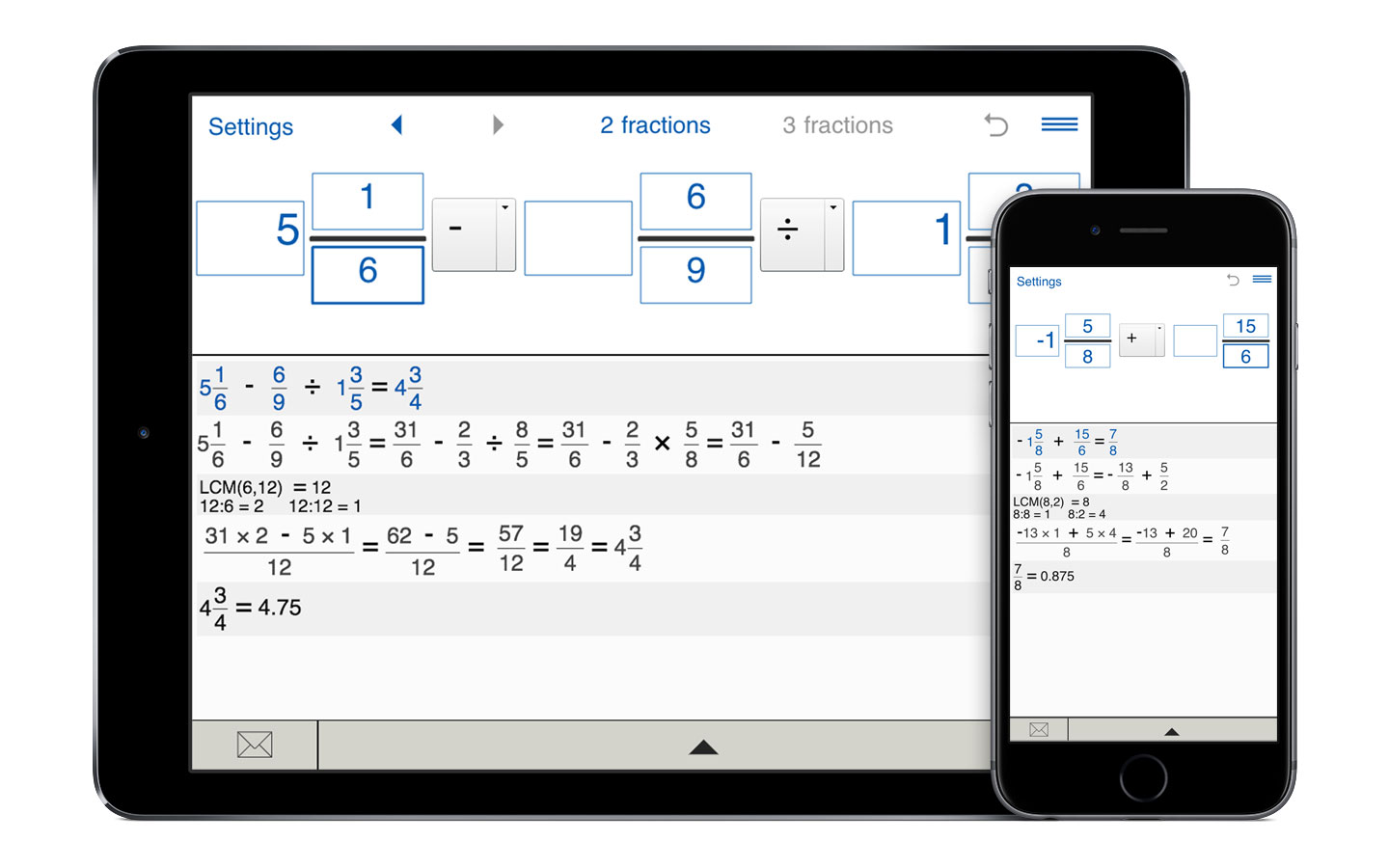# Fraction calculator with steps

Fraction calculator with steps is the best fraction app for understanding, learning and teaching fractions. It helps you validate your calculations and understand how to calculate the result.

## Calculate fractions step by step

Fraction calculator (aka fraction solver) with steps quickly solves various fraction problems, including addition, subtraction, multiplication and division of positive and negative fractions, improper fractions, whole numbers and mixed numbers. It enables you to add, subtract, multiply, and divide two and three fractions. The answer is provided in a reduced fraction.

Unlike many other fraction calculators, this Fraction calculator keeps calculation history and enables you to refer to your previous calculations. You can recall any fraction problem by simply clicking on the < (left arrow) and > (right arrow) button in the top menu.

With the history list, you can see the results of your recent calculations at a glance.

In addition, you can send all the calculation results with explanations via email.

## Fraction calculator with for Windows, Mac, iPhone, iPad, iPod and Android devices

Fraction calculator with steps has everything you need for teaching and learning fractions. It is a handy tool for adding, subtracting, multiplying or dividing fractions. Fraction calculator not just calculates the answer, but shows you how the problem was solved.
If you are a student that has difficulties with fractions or you a parent of a student who is learning fractions, then this is the app for you. Just enter the fractions, choose the operation(s) from the drop-down menu, click 'Calculate' and get the answer and step by step solution for your fraction problem.

#### Solving problems with fractions

The fraction calculator app helps you understand and practice:
- adding fractions with the same denominator
- adding fractions with different denominators
- adding two and three fractions
- subtracting fractions with the same denominator
- subtracting fractions with different denominators
- subtracting fractions and whole numbers
- subtracting mixed numbers
- adding and subtracting three fractions
- multiplying fractions
- multiplying fractions with whole numbers and mixed numbers
- multiplying mixed numbers
- dividing fractions
- dividing fractions with whole numbers
- dividing mixed numbers

Related calculators:

To perform operations on more than 3 fractions, use Fraction Calculator XL. This calculator performs operations with up to 6 fractions and shows detailed solution of the problem. You can use this Fraction Calculator XL to add, subtract, multiply and divide simple and improper fractions and mixed numbers.

If you want to reduce fractions to lowest terms, try our Simplify fractions calculator.

To compare two or three fractions, you can use the Compare fractions app. This calculator lets you compare fractions, improper fractions and mixed numbers.

If you need a calculator that performs operations with integers, fractions, decimals and mixed numbers, use Fraction Pro. The calculator supports brackets and numbers with exponents.